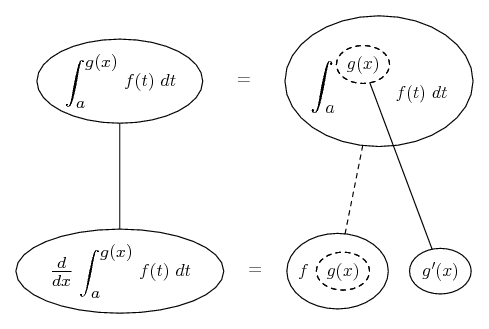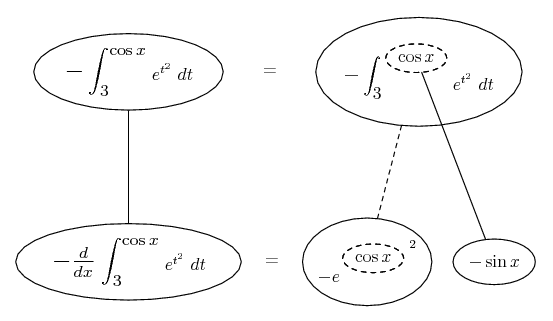# Thread: Differentiation using Second Fundamental Theorem

1. ## Differentiation using Second Fundamental Theorem

I am trying to find

$\displaystyle \frac{d}{dx} _{\cos{x}}\int^{3} e^{t^{2}}dt$

I flipped it to:

$\displaystyle \frac{d}{dx} - _{3}\int^{\cos{x}} e^{t^{2}}dt$

So I believe what I have to do is take the derivative of

$\displaystyle e^{cos^{2}{x}}$

Using the chain rule, I get

$\displaystyle e^{cos^{2}{x}} \times -2sin{x}cos{x}$

Since I flipped the integral, I multiply by negative one and get

$\displaystyle 2sin{x}cos{x}e^{cos^{2}{x}}$

But this is not the answer. Can anyone see where I went wrong? Thanks for your time

2. Just in case a picture helps...... where... is the chain rule. Straight continuous lines differentiate downwards (integrate up) with respect to x, and the straight dashed line similarly but with respect to the dashed balloon expression (the inner function of the composite which is subject to the chain rule).

So...__________________________________________
Don't integrate - balloontegrate!

Balloon Calculus; standard integrals, derivatives and methods

Balloon Calculus Drawing with LaTeX and Asymptote!

#### Search Tags

differentiation, fundamental, theorem